• 复杂网络实验1：ER随机图（matlab） 一.版本1（单纯画图,无邻接矩阵） 1.创建degree（度）一维矩阵 2.创建点位置二维矩阵 3.生成圆形的形状，把圆形分成N份，给点赋值 4.每个点之间以一定概率形成边 二.版本2...
复杂网络实验1：ER随机图（matlab）

一.版本1（单纯画图,无邻接矩阵）

1.创建degree（度）一维矩阵

2.创建点位置二维矩阵

3.生成圆形的形状，把圆形分成N份，给点赋值

4.每个点之间以一定概率形成边

二.版本2（有邻接矩阵）

鉴于一些朋友需要有邻接矩阵的版本，后面再添加有邻接矩阵的版本

由于我这里不需要邻接图的逻辑，我只要画图，所以把邻接图省略了，下面补上

由于这是比较早期的博文，下面的部分有点混乱，也不怎么想改了，给出具体的思路

具体的解析我就全放到代码部分了，可以自己看代码

1.创建邻接矩阵（N*N）

2.创建点位置矩阵(N*2)

3.安排好每个点的位置

4.先画出所有点，保持住图形

5.遍历一遍邻接矩阵

#1.刚开始的时候，邻接矩阵是全零的，这一遍遍历，就是以一定概率（程序中设置的概率是0.1）把0变成1

#2.程序中邻接表表示为adj，如果adj(2,3) = 0 ,说明节点2和节点3之间没有连边，如果是1，就是有连边存在

#3.整个邻接图是对称矩阵，就是以主对角线对称 adj(2,3) = adj (3,2)，因为是无向图

6.通过上面这一遍遍历，设定好了点与点之间的连边，再遍历一遍邻接表

#1.这一遍看到邻接表中数值是1，形成连边就行

#2.可以看到这一步是多余的，其实在上一次循环的时候，就能把线画出来，而这里的邻接表其实也没啥用

#3.就是有些朋友想要邻接表的版本而已

三.代码+解析

这里贴的代码后面用//注释，不能放入matlab运行

matlab注释要用%

简单自启动，不输入点数量，边随机重连P

N=100;                                      //100个点

p=0.1;                                       //边随机连接概率P=0.1

degree=zeros(N,1)；               //初始化每个点的度为0，N行1列

position=zeros(N,2);                  //生成N行2列零矩阵，存放点的位置

注意1：

matlab的矩阵下标从1开始

for m=1:N                                      //遍历每一行（每个点），给每个点赋值X,Y

position(m,1)=cos(m/N*2*pi)      //X是cos，在半径为1的圆上找点

position(m,2)=sin(m/N*2*pi)      //Y是sin

end

注意2：

这里我们把点分布在一个圆上,通过把2π分成N份的思路

半径为1，所以X直接取cos,Y直接取sin

figure;                                         //单纯显示一个窗口（用来显示图像）

hold on;                                     //把这个窗口保持住

plot(position（:,1），position(:,2),'o');  //画出这个圆上所有点，以空心圆的形式

注意3：

这里把这个窗口保持住的原因是，matlab每次使用plot都会默认打开一个窗口

这个默认窗口只有一个，也就是你两次调用plot命令，上一个默认图形窗口会被这次关掉

我们希望把画的东西画在一个图里，所以会使用hold on

我们先把点在图上画出来，下面要在点之间连线，所以这里要保持住

for m=1：N

for n=m+1:N                                                     //遍历每一个点，继而遍历这个点之后的点

if(rand(1,1)<p)                                             //生成一个随机数（一行一列），如果小于P（这里设置成0.1）

degree(m,1)=degree(m,1)+1;

degree(n,1)=degree(n,1)+1;                   //这两个点的度分别加1

plot(position([m,n],1),position([m,n],2));   //在第m个点和第n个点之间画一条线

end

end

end

hold off;

注意4：

这里hold off的意思就是停止保持窗口，下面我们需要展示度的窗口，这个窗口的图已经画完了

figure;                           //打开一个新窗口，不和上一个窗口冲突

hist(degree);                 //hist()是直方图的命令，默认10个值的分布，给出度分布的直方图

四.源码

N=100
p=0.1

degree=zeros(N,1);
position=zeros(N,2);

for m=1:N
position(m,1)=cos(m/N*2*pi);
position(m,2)=sin(m/N*2*pi);
end

figure('name','ER随机图');
hold on;
plot(position(:,1),position(:,2),'d')

for m=1:N
for n=m+1:N
if(rand(1,1)<p)
degree(m,1)=degree(m,1)+1;
degree(n,1)=degree(n,1)+1;
plot(position([m,n],1),position([m,n],2))
end
end
end
hold off;

figure('name','度分布直方图');
hist(degree);

有邻接矩阵版本：

N = 100  %100个点
p = 0.1  %点与点之间以0.1的概率形成连边
%可以修改上方的参数，得到不同的模型

position=zeros(N,2);    %点位置信息position,一共有N组数据，每组数据有2个信息
adj = zeros(N,N);  %创建邻接矩阵，初始化邻接矩阵全零
for m=1:N           %给每个点安排位置，围成一个圆
position(m,1)=cos(m/N*2*pi);
position(m,2)=sin(m/N*2*pi);
end

figure('name','ER随机图');
hold on;
plot(position(:,1),position(:,2),'d')

for m=1:N
for n=m+1:N
if(rand(1,1)<p)  %以0.1的概率生成边
adj(m,n)=1;  %这里两句给邻接表赋值
adj(n,m)=1;
end
end
end
for m = 1:N
for n = m+1:N
if(adj(m,n)==1)  %如果有边就画出来
plot(position([m,n],1),position([m,n],2));
end
end
end
hold off;



五.小结

在100个点，随机连边概率0.1的情况下的ER随机图与度分布直方图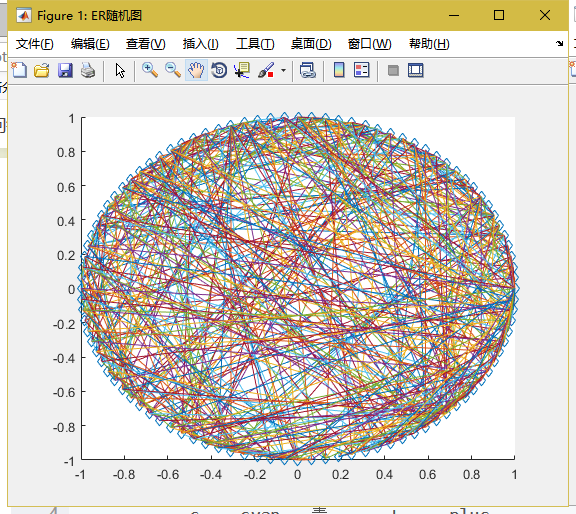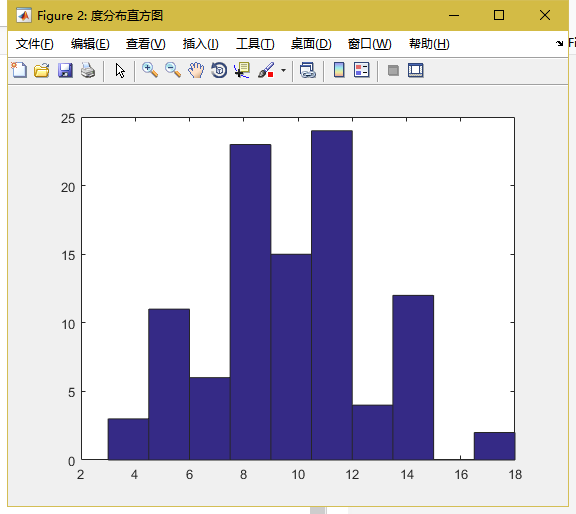展开全文ER随机图
• 复杂网络实验2：WS小世界模型（matlab） 一.思路 1.小世界模型3个参数，N为点的数目，K表示每个点左边K/2个邻居，右边K/2个邻居，一共K个邻居，P代表每条边以多少概率重连 2.首先给定这三个参数（源码是人工输入...
复杂网络实验2：WS小世界模型（matlab）

一.思路

1.小世界模型3个参数，N为点的数目，K表示每个点左边K/2个邻居，右边K/2个邻居，一共K个邻居，P代表每条边以多少概率重连

2.首先给定这三个参数（源码是人工输入，解析代码是我在代码中直接给出，通过修改代码中3个参数实现模型的变化）

3.画出以圆为轮廓的N个点

4.给出邻接矩阵A，将初始图像（每个点和邻居有边）的边存放在这里（先不画出来，就存在矩阵里，到时候边改过后再画）

5.修改边（其实是修改邻接矩阵）

6.按照邻接矩阵画出最后的图像

二.代码+解析

N=100       //给定100个点

K=4         //左右各两个邻居，一共4个邻居

p=0.1       //每条边以0.1的概率重连

这一块给定了模型的初始的3参数

angle=0:2*pi/N:2*pi-2*pi/N;   //1：狗：2的意思是，以狗为间隔，返回1和2之间的数组成的数组

//这里右边一部分不能取2*pi，不然就取了N+1个数，因为区间两边都算在内

//如果你右边取2*pi,我们是围成一个圆，这个2*pi的点其实就是0这个点

//这里只是取了100个角度，分摊2*pi这个圆

x=100*cos(angle);            //X取半径为100，角度为angle的圆上点的横坐标，注意这是个数组

y=100*sin(angle);

plot(x,y,'ro','MarkerEdgeColor','g','MarkerFaceColor','r','MarkerSize',8)  //画出这100个点

//‘ro’在绘图时，只画红色的圈，xi和yi的交点

//‘g’点的边缘取绿色

//‘r’点的内部取红色

//‘8’标记大小为8

hold on;      //图像保持住，画出点，等等还要画线

到这里把点画好了

A=zeros(N);            //生成一个N行N列的0矩阵赋值给A，当做初始化的邻接矩阵

for i=1:N

for j=i+1:i+k/2     //每次取当前点，并且问候右边两个邻居，给他们握手（互相加1）

jj=j;               //j赋值给jj

if j>N

jj=mod(j,N);   //如果j的右边邻居超过N，要从0开始找他的邻居

end

A(i,jj)=1;

A(jj,i)=1;            //邻接矩阵表现成我和这个邻居各加1，其实是个对称矩阵，在操作右上角的时候顺带操作左下角

end

end

//为什么要把j给jj? jj是处理过的j,因为j有时候会超过N，要转一圈

//这个时候我们的基础图完成了，接下来开始随机重连

//把图存放在邻接矩阵A中

for i=1:N

for j=i+1:i+k/2         //还是和上面一样我每次找到右边的邻居

jj=j;

if j>N

jj=mod(j,N);        //如果j可能是假邻居的话，那么jj必定是真的邻居

end

p1=rand(1,1);          //生成一个1行1列的数赋值给p1

if p1<p                 //p1小于之前设定的p,说明以0.1的概率，需要修改边

A(i,jj)=0；

A(jj,i)=0;

A(i,i)=inf;           //怕等等自己和自己连，取值无穷

a=find(A(i,:)==0);    //找到A中i行所有等于0的，就是没和i相连的点，a数组存放着所有和当前点没有连接的点

rand_data=randi([1,length(a)],1,1);    //1行1列，随机数，随机找一列

jjj=a(rand_data);

A(i,jjj)=1;

A(jjj,i)=1;

A(i,i)=0;   //从inf恢复

end

end

end

for i=1:N

for j=i+1:N            //遍历邻接矩阵的右上角

if A(i,j)~=0            //如果点i与点j之间有边（邻接矩阵中A（i,j）为1）

plot([x(i),x(j)],[y[i],y[j]],'linewidth',1.2);    //在(x(i),y(i)),(x(j),y(j))之前生成边，线宽1.2

hold on;

end

end

end

axis equal;             // 使横轴，纵轴相等

hold off;

三.源码

N=input('请输入最近邻耦合网络中节点的总数N:');

K=input('请输入最近邻耦合网络中每个节点的邻居数K:');

if K>floor(N-1)|mod(K,2)~=0;
disp('参数输入错误：K值必须是小于网络节点总数且为偶数的整数');
return ;
end

angle=0:2*pi./N:2*pi-2*pi/N;
angle=0:2*pi/N:2*pi-2*pi/N;
x=100*sin(angle);
y=100*cos(angle);
plot(x,y,'ro','MarkerEdgeColor','g','MarkerFaceColor','r','MarkerSize',8);

hold on;
A=zeros(N);
for i=1:N
for j=i+1:i+K/2
jj=j;
if j>N
jj=mod(j,N);
end
A(i,jj)=1;
A(jj,i)=1;
end
end

%WS小世界网络的代码
p=input('请输入随机化重连的概率p:');
for i=1:N
for j=i+1:i+K/2
jj=j;
if j>N
jj=mod(j,N);
end
p1=rand(1,1);
if p1<p
A(i,jj)=0;A(jj,i)=0;A(i,i)=inf;a=find(A(i,:)==0);
rand_data=randi([1,length(a)],1,1);
jjj=a(rand_data);A(i,jjj)=1;A(jjj,i)=1;A(i,i)=0;
end
end
end

for i=1:N
for j=i+1:N
if A(i,j)~=0
plot([x(i),x(j)],[y(i),y(j)],'linewidth',1.2);
hold on;
end
end
end
axis equal;
hold off

四.小结

生成的小世界图像如下图所示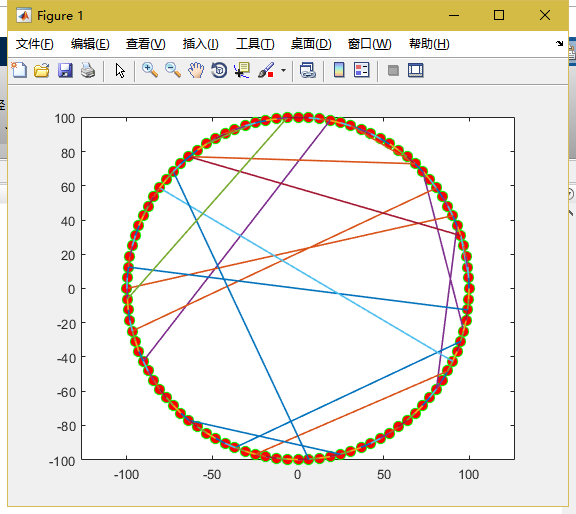展开全文小世界模型
• 一、SIR模型简介 在典型的传染病模型中，种群（Population）内的N个个体的状态可分为如下几类： (1)、易染状态S(Susceptible),即健康状态，可被感染的个体。 (2)、感染状态I(Infected),处于感染状态的个体还能够...
一、SIR模型简介

在典型的传染病模型中，种群（Population）内的N个个体的状态可分为如下几类：

(1)、易染状态S(Susceptible),即健康状态，可被感染的个体。

(2)、感染状态I(Infected),处于感染状态的个体还能够感染将康状态的个体。

(3)、移除状态R(Removed,Refractory or Recovered)，也称为免疫状态和恢复状态。一个个体经历过一个完整的感染周期后，该个体就不再被感染，因此就可以不再考虑该个体。

另外还有病人的日接触率λ，日治愈率μ

这个λ是针对于病人而言的，代表了一个病人接触多少个人。而可接触的人包括除自己以外的种群中的所有人。

tips:

1.初始时刻，只有少数个体处于感染状态，其他都是易染状态。

2.假设病毒的时间尺度远小于个体生命周期，从而不考虑个体的出生和自然死亡。

3.一个基本假设是完全混合（Fully mixed）,也就是说一个个体与其他个体接触的机会均等。

二、模型中涉及的方程

1.S(t),I(t),R(t),N(t),N

S(t)的意思是第t天健康个体的数量，I(t)是第t天感染个体的数量，R(t)是第t天免疫个体的数量

N(t)是整个种群的数量，在假设情况下固定不变为N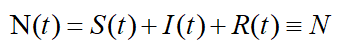2.s(t),i(t),r(t)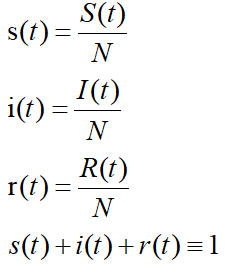由上方公式可以看出，s(t)的意思是t时刻健康个体占总个体的比例

3.关于S(t),I(t),R(t)的微分公式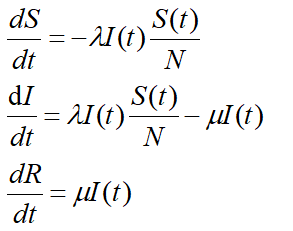其中S随时间的变化率是这样理解的：

λ作用于S(t)和I(t),是一个病人的日接触率，这个病人可以接触健康人，也可以接触病人，但是接触病人不会导致S有变化，所以有效的变化是这个病人接触健康人。

如果λ等于2，也就是说一个病人每天接触2个人，这两个人是不是病人不知道。

如果λ等于0.5，也就是说一个病人每天接触0.5个人（有0.5几率去接触人）

那么λ×I(t)就是所有被接触的人的数量，要把里面有效的人拿出来

有效的人的概率是S(t)/N。

这样应该能理解了吧，我理解这个公式用了好久好久，简直是一个傻吊。

网上有的文章i(t)和I(t)不分，导致理解公式特别困难。

最后作图都是用的i(t)，s(t),r(t)随t的变化的图像，所以纵坐标是一个百分比。

4.关于s(t),i(t),r(t)的微分公式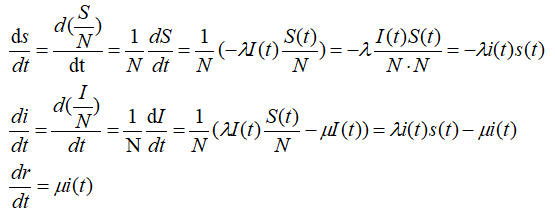这里细致地做了微分公式的推导，方便大家理解

三、具体模型

1.sir.m 脚本

function y=sir(t,x)
a=0.8;  %感染率0.8
b=0.2;  %治愈率0.2
y=[-a*x(1)*x(2),a*x(1)*x(2)-b*x(2),b*x(2)]';
%s变化率,i变化率，r变化率
%通过这三个微分公式，求出s,i,r随着t的变化图像

2.实际运行脚本 sirrum.m

[t,x]=ode45('sir',[0,50],[0.97 0.02 0.01]);
%ode45参数：1.函数句柄or函数名 2.t的取值 3.3个y的初始值
%ode45是用来求解常微分函数的方法
%原问题只知道变化率函数，这里求解原函数，用到该方法
[t,x]   %不加封号，作输出用
plot(t,x(:,1),'-',t,x(:,2),'*',t,x(:,3),'+')

四、小结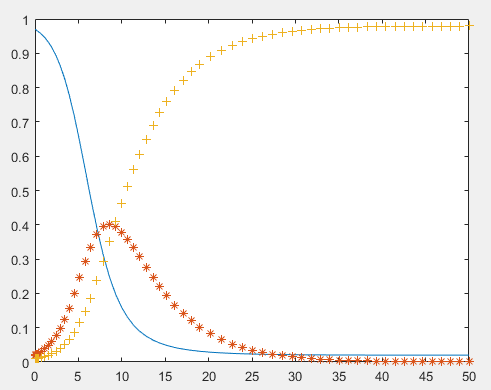搞了一下午才把那个公式看懂，网上的公式不好好说明清楚，很具有迷惑性，可能他们也没了解这个小写函数大写函数的区别。

在豆瓣《猜想与反驳》一书底下评论中看到一句有趣的话，摘录如下：

每个知识分子都有一个很特殊的责任。

他拥有学习的特权和机会。

作为回报，他对于同胞（或对于社会）有责任尽可能简单，清楚，谦虚地描述他的研究结果。

知识分子所做的最糟的事情(主要罪过)是，

试图对同胞自命为伟大的预言家，给他们留下令人迷惑的哲学的印象。

任何不能简单，清楚地讲话的人最应住口，继续下写功夫，直至能这样做为止。

所以，有什么问题都可以问我，如果我能帮你的话，虽然我也是一只很菜的菜鸟........


展开全文matlab
• m_original:未增长前的网络节点个数 m_add:每次引入新节点时新生成的边数 m_after_growth:增长后的网络规模 pp:初始网络节点的连接选择 pp=1 节点均孤立 pp=2 节点间构成完全图 pp=3 随机连接一些边(随机图) ...
一.思路

1.首先确定BA模型中最重要的4个参数

m_original:未增长前的网络节点个数

m_add:每次引入新节点时 新生成的边数

m_after_growth:增长后的网络规模

pp:初始网络节点的连接选择

pp=1 节点均孤立  pp=2 节点间构成完全图  pp=3 随机连接一些边(随机图)

代码中这些参数都在最前面给出，可以通过修改代码来修改模型

2.在100*100的空间中生成m_original个点

3.通过pp值，初始化邻接矩阵A

4.最后生成节点，每个节点和之前m_add个节点相连

网络科学导论的BA模型没有考虑孤立节点，但是我感觉孤立节点也应该有几率被连接吧，总得给人家一点机会吧

不考虑孤立节点，那么一个点的连接概率按照该点的度数/总度数可以得到，因为不是孤立节点就有连边，就有度数，就有机会能够连接

但是考虑孤立节点，没有度数，再按上方的公式给孤立节点分配连接概率，是不妥的

这里考虑的模型是我思考出的存在孤立节点的BA模型的一个解决方案

这里有三个值得讨论的点

①.连接概率的设置

首先考虑到稀疏图中这样的场景，一共10个点，只有一条边，也就是只有两个点度数为1，其余8个点度数都是0

这个时候，你如果用上方的公式：点度数/总度数 来分配概率，那么这8个点都没机会连接，其余两个点的连接概率是1/2

这显然不合理，因为，这些边其实相差不大，甚至可以说基本都一样，应该都有机会能够连接。

这里我想出的策略是

每个点的度数：1+原度数

总度数为：原来总度数+总点数（每个点多加一个点数）

这样每个孤立点也有机会进行连接，度数越大连接概率也越大，不影响优先连接机制

②.连接概率存在的情况下，怎么做到随机连边

不是说你概率越大，我就连接，这里还是使用一个随机数的方法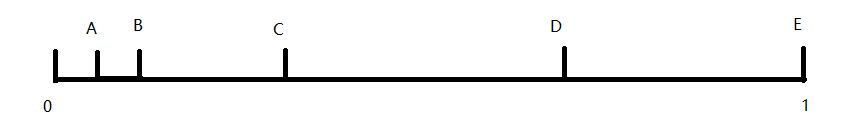如上图所示，一共ABCDE5个点，A的连接概率体现在0-A这一个区间，也就是A占据了0-A，说明A的连接概率一般

D的连接概率体现在C-D这个区间，也就是D占据了C-D，说明D的连接概率最大

那么我们怎么做到用上面这样的策略达到目的呢？

概率叠加

假设A的连接概率为0.1,B为0.1,C为0.2，D为0.4，E为0.2

那么我们让A就是0.1，B在0.1+0.1=0.2，C在0.1+0.1+0.2=0.4，以此类推

然后每次要选一个点进行连接的时候，取一个0-1之间的随机数，看掉落在这5个区间中的哪一个，然后取这个区间的右端端点所代表的节点进行连接，因为这个区间是他的主场（之前说过哪个区间被哪个节点占据）

③怎么保证每次增加一个节点就增加m_add条边？怎么保证不重复连接

这里我在网上的模型中没找到，我使用了visit数组的概念

当你这个节点已经连接过，那么visit数组值1，下次分配连接概率置0

当然计算总度数的时候这个点的度数就不考虑了

重新给每个点分配一遍概率，进行概率叠加，再进行连接

因为连接过的点连接概率置0，所以不会重复连接，也能够起到随机连接，优先连接的目的

附：模型设定

初始状态有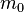个节点

增长原则：每次加入一个节点i （加入时间记为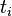）, 每个节点的加入带来m条边，2m个度的增加

其中老节点分到的度数是m，新加入的那一个节点分到的度数为m

那么到时间t的时候，网络的总节点数是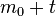，网络的总度数为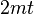。

优先链接原则：每一次从m条边中占有一条边的概率正比于节点的度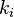那么显然，加入的越早（越小）越容易获得更多的链接数。

从时间0开始，每一个时间步系统中的节点度是不断增加的。

二.代码＋解析

1.第一部分，四个参数

m_original=100;                   //未增长前网络节点个数m_originalm_add=3;                             //每次添加一个点增加的边数m_addm_after_growth=1000;         //增长后的网络规模m_after_growthpp=1;

2.得到m_original个点的横纵坐标(初始网络的横纵坐标)

x=100*rand(1,m_original);
y=100*rand(1,m_original);

rand(1,n)是生成一行n列的列向量，其中每个数的值是0-1,再乘上100，相当于把点分布到100*100的二维空间中

3.通过pp值得到初始网络（初始邻接矩阵A）的状态

A=zeros(m_original);                    //初始化邻接矩阵A为全0矩阵
switch pp                                     //通过选择pp，确定A的初始状态
case 1                                      //节点均孤立
A=zeros(m_original);
case 2                                      //完全图
A=ones(m_original);
case 3                                     //随机图
for i=1:m_original
for j=i+1:m_original         //操纵上半角矩阵
p=rand(1,1);               //生成0-1随机数
if p>0.5                       //以1/2的概率生成边
A(i,j)=1;
A(j,i)=1;
end
end
end
end

4.生成增加的节点和边

for k=m_original+1:m_after_growth     //一共生成m_after_growth-m_original+1个节点
M=k-1;                                             //当前要生成第K个节点，那么针对的图的规模就是k-1
p=zeros(1,M);                                  //初始化每个点的连接概率为0

x_now=100*rand(1,1);                    //随机生成第K个节点的坐标
y_now=100*rand(1,1);
x(k)=x_now;
y(k)=y_now;

for i=1:M
p(i)=(length(find(A(i,:)==1))+1)/(length(find(A==1))+M);
end                                             //这里就是前面说的修正孤立节点连接概率为0的方法

p                                                  //不加封号的都是为了在控制台显示出来，看看p的生成情况
pp=cumsum(p);                           //这里就是上面说的叠加概率
pp

备注：对于cumsum（）方法，有下方测试帮助理解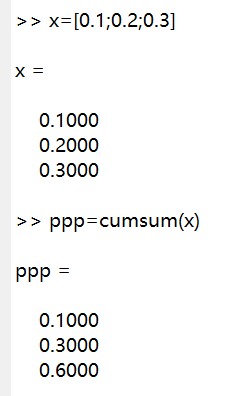visit=zeros(1,M);                   //初始化访问数组为全0数组（1行M列）
for i=1:m_add                                       //开始生成m_add条边
random_data=rand(1,1);
random_data
aa=find(pp>=random_data); jj=aa(1);       //通过上方介绍的叠加概率+随机数的方法，得到随机连边 备注：find函数返回括号内“满足条件的pp的数组的下标”的数组,jj取这个数组的第一个元素，对应占领这块区域的点

A(k,jj)=1;
A(jj,k)=1;

visit(jj)=1;      //标记访问
visit               //显示访问情况

degree=zeros(1,M);      //给出度数组来记录变化后的度值，以达到访问过度数假装变成0的目的
total_degree=0;            //总度数
for ii=1:M
if visit(ii)==1
p(ii)=0;
degree(ii)=0;
else
degree(ii)=length(A(i,:)==1)+1;         //如果访问过度数为0，没有就照原来的方法
end

total_degree=total_degree+degree(ii);   //总度数计算
end

for iii=1:M
p(iii)=degree(iii)/total_degree;            //新一轮的p数组计算
end
p
pp=cumsum(p);                                     //新一轮的叠加概率计算
pp
end
end

A
plot(x,y,'ro','MarkerEdgeColor','g','MarkerFaceColor','r','MarkerSize',8);         //画出点
hold on;
for i=1:m_after_growth
for j=i+1:m_after_growth                                                    //按邻接矩阵上三角矩阵画出连线
if A(i,j)~=0                                                     //~=0是不等于0的意思，就是有边，就画出来
plot([x(i),x(j)],[y(i),y(j)],'linewidth',1.2);
end
end
end

axis equal;                 //横纵坐标相等
hold off;

三.源码

m_original=10;
m_add=4;
m_after_growth=11;
pp=1;

x=100*rand(1,m_original);
y=100*rand(1,m_original);

A=zeros(m_original);
switch pp
case 1
A=zeros(m_original);
case 2
A=ones(m_original);
case 3
for i=1:m_original
for j=i+1:m_original
p=rand(1,1);
if p>0.5
A(i,j)=1;
A(j,i)=1;
end
end
end
end

for k=m_original+1:m_after_growth
M=k-1;
p=zeros(1,M);

x_now=100*rand(1,1);
y_now=100*rand(1,1);
x(k)=x_now;
y(k)=y_now;

for i=1:M
p(i)=(length(find(A(i,:)==1))+1)/(length(find(A==1))+M);
end
p
pp=cumsum(p);
pp
visit=zeros(1,M);
for i=1:m_add
random_data=rand(1,1);
random_data
aa=find(pp>=random_data); jj=aa(1);

A(k,jj)=1;
A(jj,k)=1;
visit(jj)=1;
visit
degree=zeros(1,M);
total_degree=0;
for ii=1:M
if visit(ii)==1
p(ii)=0;
degree(ii)=0;
else
degree(ii)=length(A(i,:)==1)+1;
end
total_degree=total_degree+degree(ii);
end
for iii=1:M
p(iii)=degree(iii)/total_degree;
end
p
pp=cumsum(p);
pp
end
end

A
plot(x,y,'ro','MarkerEdgeColor','g','MarkerFaceColor','r','MarkerSize',8);
hold on;
for i=1:m_after_growth
for j=i+1:m_after_growth
if A(i,j)~=0
plot([x(i),x(j)],[y(i),y(j)],'linewidth',1.2);
end
end
end

axis equal;
hold off;



四.小结

为了有直观的体验，可以把pp设置为1，即完全孤立图
初始点数可以设置成10，增长后的规模可以设置成11，每次连接4个点
之后再慢慢增大网络规模进行测试，验证是否每增加一个点就能有这么多条边

最后设置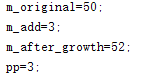得到的图像如下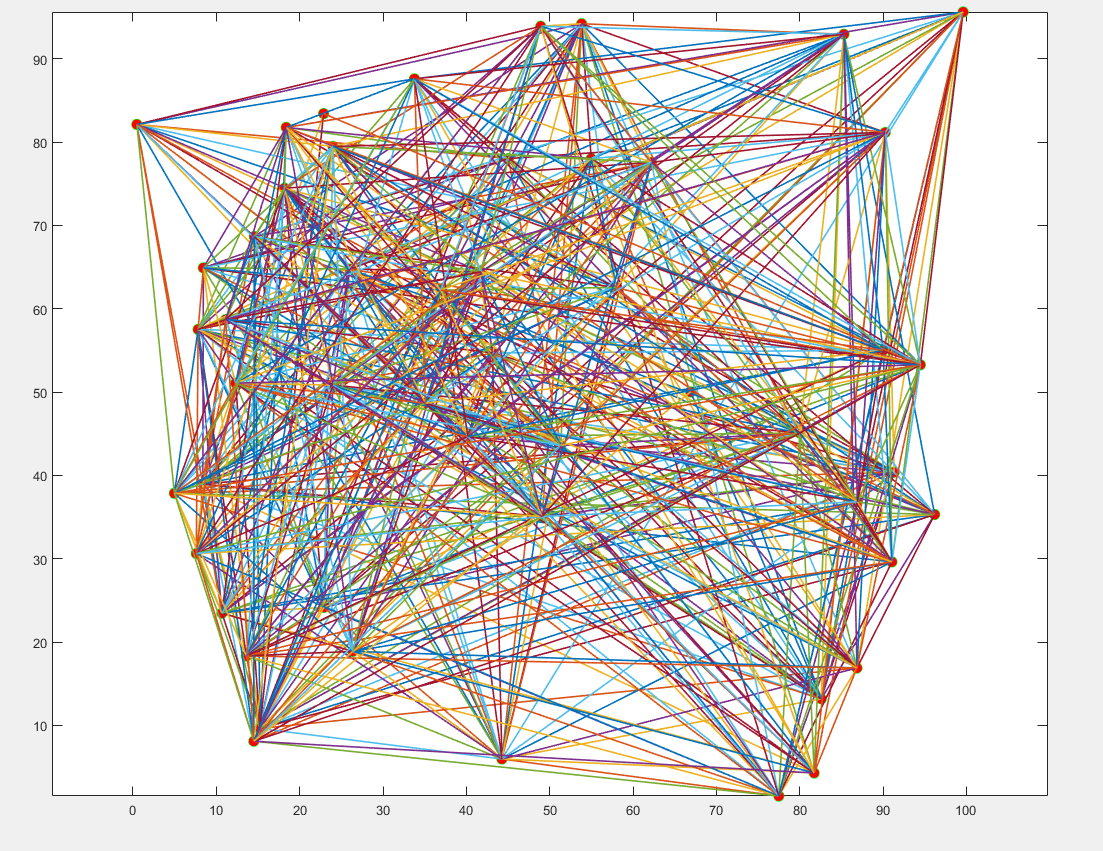展开全文matlab
• 可以证明：从网络中随机选出一个节点，顺着有向边行走K步之后，位于节点i的概率， 等于运用pagerank算法k步后得到的节点i的PR值。 这个结论很厉害，写在书上的应该错不了，书上也没给出证明，就先把这个结论当个...pagerank matlab
• 一、简介 1.定义 为了理解非平衡系统的分簇，传递和相变 vicsek提出了自驱动粒子模型(Self-Propelled Particle,SPP)模型 Vicsek模型是一种用于描述“Active matter”的数学模型 该模型在较高的粒子密度或较低的...matlab quiver
• 一、背景知识 ... 相关内容在我上一篇pagerank算法中提到了，可以查看。 二、LeaderRank算法介绍 ...这个新的网络成了一个强连通网络，修复了基本的pagerank算法的一些问题。 刚开始所有页面的...matlab
• 一.思路 一维元胞自动机 一维元胞自动机的意思是，当前元胞的状态，只能被左右元胞的状态影响，而不能被上下元胞影响（能上下就是二维了） 为什么普通一维元胞自动机（只有当前元胞和左右两个邻居）规则有256个？...一维元胞自动机
• 一.定义 1.二维元胞自动机 所谓二维元胞自动机，就是当前元胞的状态不但可以由左右元胞决定，还能被上下元胞，甚至是其他二维平面内的元胞状态决定。 2.生命游戏（game of life） 生命游戏是英国数学家约翰·...二维元胞自动机 生命游戏
•研究论文
• 一个实现的复杂指令系统模型机 的实验报告 内有源码
• 复杂传染 Complex Contagions是一个python库，可以在嘈杂的几何网络上运行简单的阈值模型（Watts Threshold模型）。 然后，可以使用gudhi python软件包对从第一个激活矩阵T（i-> j）获得的距离矩阵进行持久的同源性...
• 计算机网络是很复杂的一个事务，进行计算机网络实验是为了提升用户使用需求。下面是学习啦带来的计算机网络实验的心得体会，仅供参考。计算机网络实验心得体会篇一计算机网络课程的实验不同于以前做过的C语言上机...
• 摘要| 基于图论的复杂网络定量分析的最新进展已迅速转化为大脑网络组织的研究。 大脑的结构和功能系统具有复杂的网络功能，例如小世界拓扑，高度连接的中心和模块性，无论是在人类神经成像的全脑范围内，还是在非...聚类算法 深度学习 大数据 机器学习 人工智能
• 关于计算机网络的一些实际操作，从最基础的实验开始，一直到复杂实验都有，看完后基本可以z掌握计算机网络的知识点。并且其中包括了计算机网络中的大多数实验实例。
• , 网络技术和概念通常比较复杂，即使通过详细的描述和深思熟虑的例子，也往往难以解释清楚。利用软件工具仿真网络的功能，为网络专业人员和学生创建可视化环境以展示网络是如何工作的，这是深入理解网络技术和概念的...
• 针对传统自相关验证方法在短时间内验证主机数量少的情况，提出了基于复杂网络理论的空间网络属性安全验证方法研究。依据空间网络现实、虚拟和社会的相关属性，结合复杂网络理论构建空间网络属性模型。根据该模型，...
• Large Network Dataset Collection）实验数据集 主要包含以下数据集 Social networks : online social networks, edges represent interactions between peopleNetworks with ground-truth ...
• 首先基于复杂网络理论构建了邮件网络的拓扑模型，分析了邮件网络的拓扑特性，在此基础上提出了一种基于点权和边权（Weight of Node and Edge， WNE）的邮件网络搜索策略。最后通过实验验证了该策略在搜索代价上优于...
• 传统协议识别技术多以单网络流为识别手段，不能应对复杂网络应用多服务、多协议等特性，因此在面对复杂网络应用识别时严重失效。...实验结果表明，流感知模型能有效识别复杂网络应用，具有良好的识别效果。行为分析
• 关于复杂网络的嵌入（embedding）陆陆续续学习了近半年之多，在此总结一下相关的基本方法和实验。 Node2vec实验 Node2vec实验模拟生成了一个简易的邻接矩阵，将其可视化如图1所示，用Node2vec方法将其嵌入到低维向量...
• 复杂网络的无害性是一个重要问题，因为无标度网络的行为不同于指数网络。 根据网络的结构特点，我们提出了两种新的攻击策略，分别称为IDB（初始程度和中间性）和RDB（重新计算程度和中间性）。 这些策略源自ID（初始...
• 针对在线社会网络中用户间的关系存在多种关系复合的情况，采用多子网...最后通过仿真实验，得出了基于多子网复合复杂网络模型的网络节点个数、网络边数、网络平均最短路径等网络特征和同等实际网络的网络特征较为吻合。
• 链路预测是复杂网络中研究缺失连边和未来形成连边的重要组成部分，当前基于网络结构的链路预测方法成果丰富，而基于复杂网络动力学模型的链路预测研究较少。针对无权无向网络，首先构建了复杂网络动力学模型，然后给...链路预测 网络动力学
• 选取复杂网络特征变量—聚集系数为研究目标，通过数学推导与证明，清晰描述了k-核与聚集系数的关联性。通过仿真实验证明，随着k-核的不断解析、k值的不断增加，网络聚集系数亦呈现逐步增加的趋势。该结论为k-核解析...
• 04 小世界网络 4.1小世界特性 4.2WS模型 4.3小世界网络中的导航 4.1小世界特性 Milgram小世界实验1 信件传递实验 六度分离理论 在线社会网络随事件推移，人与人的间隔也在下降。 “小”的含义 平均距离小：...
• 社团结构是复杂网络最普遍和最重要的属性之一，复杂网络的社团区划研究就是要合理地划分出复杂网络中真实存在的社团结构。主要将超网络的思想及理论方法应用于复杂网络的社团区划研究，针对当前GN算法的一些不足，从...GN算法
• 提出一种延迟修复的网络修复模型，该模型考虑了网络打击和修复所需要的实际时间比例，并在不同复杂网络拓扑下针对随机打击和故意打击这两种打击模式设计了四种不同的修复策略，模拟实验结果显示在随机网络下，采用...
• 论文研究-Caveman网络及其在复杂网络熵分析中的应用.pdf, 熵可以有效反映复杂系统内网络结构的异质性.针对熵指标在刻画网络全局异构上是否适用这一问题，目前仍缺少用以......